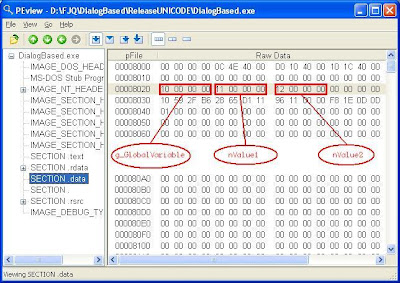## Thursday, June 19, 2008

### How Local Static Variable Is Implemented in vc++

As every one knows, static variables are initialized only ones, even though it is declared in a function. In this post, I will explain how the compiler implements this.

OK let start with the storage of the static variables. Where do you think a static variable declared inside a function is stored? If your answer is on the stack, you are wrong. All static and global variables are stored in a section called ".data" in the exe. For example check the below code.
`int g_GlobalVariable = 0x10;static int nValue1 = 0x11;void main(){     static int nValue2 = 0x12;     // Take the address     int *pglobal = &g_GlobalVariable;     int *pValue1 = &nValue1;     int *pValue2 = &nValue2;}`
When executed, the values of pglobal, pValue1 and pValue2 were 0x408020, 0x408024 and 0x408028 respectively. Which means, all the three variables were stored in three consecutive locations. In the PE view we can see these variables stored in the ".data" section.You can see that the "nValue2" variable is initialized at the compile time itself. so no instruction is generated by the compiler for a statement like static int nValue2 = 0x12;

So compiler did a nice job by setting the value to variable at the compile time itself. But that was a C type object. What if we declare a static C++ object inside a function. This time the compiler cannot initialize the variable at the compile time because the constructor need to be invoked to initialize a C++ object. Consider the below code

`class TestClass{public:  TestClass()  {    m_nMemeberVar = 10;  }  int m_nMemeberVar;};TestClass& AFunction(){  static TestClass cppObject;  return cppObject;}`

In the above code, the AFunction() function creates a static object of class TestClass and as you know the cppObject will be initialized only once. How does the compiler manages to do it? Well answer is compiler keeps a flag!!! I.e when the compiler sees a c++, static object is declared inside a function, it will create a global byte variable with value 0 and some addition instruction such that the constructor of the object will be called only if the value of the byte variable is 0 and it also set the value of the byte variable as 1. So from the next time onwards, the constructor will not be called. Below is the dis assembly for the statement static TestClass cppObject.

`static TestClass cppObject;`
`// Zero out the eax register00401E79   xor         eax,eax`
`// Copy the flag variable to al register00401E7B   mov         al,[`AFunction'::`2'::\$S230 (00408104)]00401E80   and         eax,1`
`// Check eax equal to zero00401E83   test        eax,eax`
`// If it is not zero, go to end.00401E85   jne         AFunction+3Dh (00401ead)`
`// Copy the flag variable to the cl register00401E87   mov         cl,byte ptr [`AFunction'::`2'::\$S230 (00408104)]`
`// cl = 100401E8D   or          cl,1`
`// Copy back the content of cl register to the flag variable00401E90   mov         byte ptr [`AFunction'::`2'::\$S230 (00408104)],cl`
`// Call the constructor of cppObject00401E96   mov         ecx,offset theApp+0C8h (00408100)00401E9B   call        TestClass::TestClass (00401ec0)`